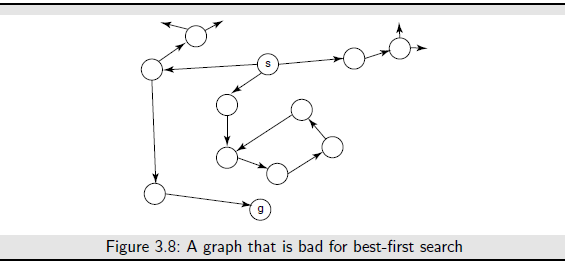Heuristic Search: All of the search methods in the preceding section are uninformed in that they did not take into account the goal. They do not use any information about where they are trying to get to unless they happen to stumble on a goal. One form of heuristic information about which nodes seem the most promising is a heuristic function h(n), which takes a node n and returns a non-negative real number that is an estimate of the path cost from node n to a goal node. The function h(n) is an underestimate if h(n) is less than or equal to the actual cost of a lowest-cost path from node n to a goal. The heuristic function is a way to inform the search about the direction to a goal. It provides an informed way to guess which neighbor of a node will lead to a goal.
There is nothing magical about a heuristic function. It must use only information that can be readily obtained about a node. Typically a trade-off exists between the amount of work it takes to derive a heuristic value for a node andhow accurately the heuristic value of a node measures the actual path cost from the node to a goal.
A standard way to derive a heuristic function is to solve a simpler problem and to use the actual cost in the simplified problem as the heuristic function of the original problem.

The h function can be extended to be applicable to (non-empty) paths. The heuristic value of a path is the heuristic value of the node at the end of the path. That is:
h(no, . . . , nk) = h(nk)
A simple use of a heuristic function is to order the neighbors that are added to the stack representing the frontier in depth-first search. The neighbors can be added to the frontier so that the best neighbor is selected first. This is known as heuristic depth-first search. This search chooses the locally best path, but it explores all paths from the selected path before it selects another path. Although it is often used, it suffers from the problems of depth-fist search. Another way to use a heuristic function is to always select a path on the frontier with the lowest heuristic value. This is called best-first search. Itusually does not work very well; it can follow paths that look promising because they are close to the goal, but the costs of the paths may keep increasing.